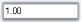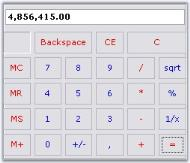# How to Customize the Calculator Display Text Area to Use NumberGroupSeparator

The Calculator control by default does not allow the use of NumberGroupSeparator like in DoubleTextBox.So to achieve this we need to derive the Calculator control and override the CreateCalculatorDisplayBox() method.

``````private CalculatorAdv calculatorControl1;
{
{
}
protected override void CreateCalculatorDisplayBox()
{
Syncfusion.Windows.Forms.Tools.DoubleTextBox dtb = new Syncfusion.Windows.Forms.Tools.DoubleTextBox();
dtb.NumberGroupSeparator = ",";
this.textCalculatorBox = dtb; //Changing the TextBox to DoubleTextBox
}
}``````
``````Private calculatorControl1 As CalculatorAdv